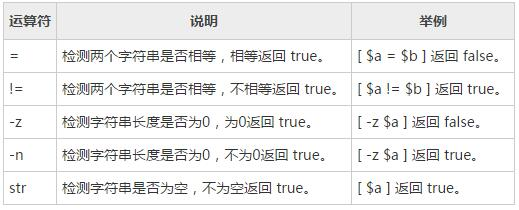# 字符串运算符

``````#!/bin/sh
a="abc"
b="efg"
if [ \$a = \$b ]
then
echo "\$a = \$b : a is equal to b"
else
echo "\$a = \$b: a is not equal to b"
fi
if [ \$a != \$b ]
then
echo "\$a != \$b : a is not equal to b"
else
echo "\$a != \$b: a is equal to b"
fi
if [ -z \$a ]
then
echo "-z \$a : string length is zero"
else
echo "-z \$a : string length is not zero"
fi
if [ -n \$a ]
then
echo "-n \$a : string length is not zero"
else
echo "-n \$a : string length is zero"
fi
if [ \$a ]
then
echo "\$a : string is not empty"
else
echo "\$a : string is empty"
fi
``````

``````abc = efg: a is not equal to b
abc != efg : a is not equal to b
-z abc : string length is not zero
-n abc : string length is not zero
abc : string is not empty
``````